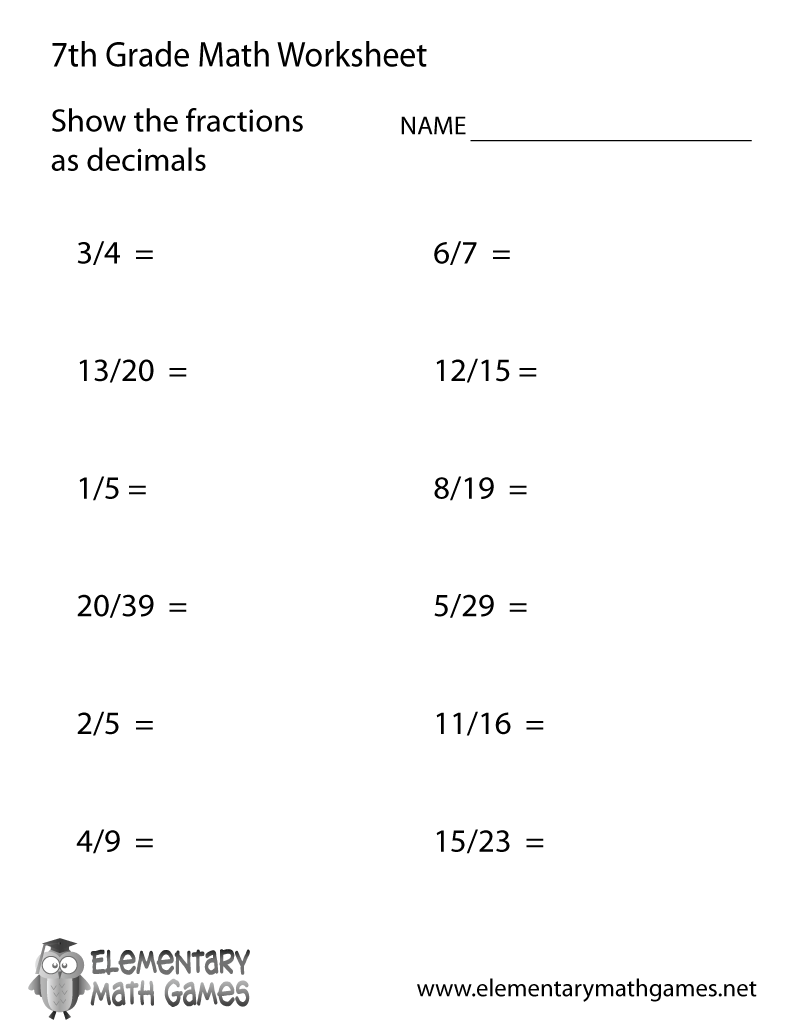# Seventh Grade Fractions and Decimals Worksheet## Learn and Practice Fractions and Decimals

This seventh grade math worksheet is a great way for kids to practice converting decimals into fractions. Seventh graders have 12 math problems that need to be solved with a fraction.

Here are the two versions of this free seventh grade math worksheet:

Seventh Grade Fractions and Decimals Worksheet PDF – Download, Best Quality
Printable Seventh Grade Fractions and Decimals Worksheet – Print Directly in the Browser

Teachers and parents can download the answers to this math worksheet here:

7th Grade Fractions and Decimals Worksheet Answers – Check Answers Quickly

### Printing is Easy

The most important aspect of our math website is usability. We want to make it easy for students, parents, and math teachers to use our educational materials. There are two colorful icons above this seventh grade worksheet. The icon labeled “Download” will prompt you to download the PDF version of this free elementary math worksheet. Printing the PDF will produce the best results. The next icon is labeled “Print”. Selecting this will take you to another web page where the fractions and decimals worksheet has been isolated so that you can print the worksheet inside of your browser.